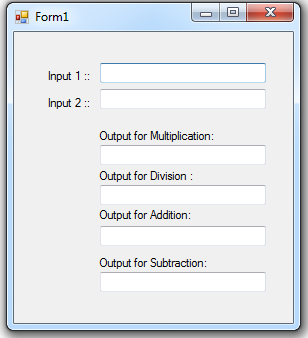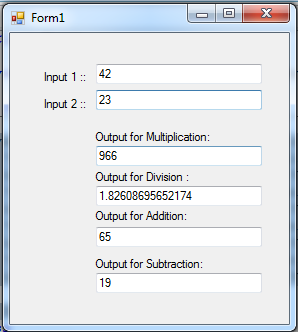# TextBox Control

One of the most common control used in visual basic is a text box. The main purpose of textbox is that, it allows user to input text information to be used by the program. By default, text box takes a single line of text. But using visual basic, you can set the property to accept a multiple line of text and even with a scroll bar. This time, open visual basic and create a new project called “Textbox”. Then drag a six text box and six label from the toolbox. And arrange all the objects that look like as shown below.What we're doing here is a Calculator for Multiplication, Division, Addition and Subtraction, that when the user type the value for the Input 1 and during the encoding the values in Input 2 the program will automatically compute for each operator and display the output in the corresponding text box. To do this add the following code: In this code below, we use TextChanged event that triggered when there is any change in the textbox. Then it will automatically compute for our different operators.
`Private Sub txtinput2_TextChanged(ByVal sender As System.Object, ByVal e As System.EventArgs) Handles txtinput2.TextChanged	'will do the computation for multiplication	txtmul.Text = Val(txtinput1.Text) * Val(txtinput2.Text)	'will do the computation for Division	txtdiv.Text = Val(txtinput1.Text) / Val(txtinput2.Text)	'will do the computation for Addition	txtadd.Text = Val(txtinput1.Text) + Val(txtinput2.Text)	'will do the computation for Subtraction	txtsub.Text = Val(txtinput1.Text) - Val(txtinput2.Text) End Sub`
When executing the program, it will give an output that look likes as shown below.